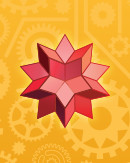# Computational Knowledge of Continued FractionsComments Off

Mathematics has many faces. It deals with diverse objects such as integers, points and lines, equations, graphs, categories, thousands of different spaces (from R3 to Hilbert, Banach, Fréchet, …), and so on. Mathematics can be constructive or just prove the existence of certain structures. Wolfram|Alpha has made a good fraction of computable constructive mathematics freely available to everyone: from line through (2,3) and (4, 5) to Fréchet derivative of (integrate exp(-f(x)^2) dx from -inf to inf) wrt f(y) to fractional derivative of ln(z). Independent of the concrete objects studied, mathematical results always come in the form of axioms, definitions, theorems, lemmas, corollaries, conjectures, proofs, algorithms, identities, and formulas, supplemented by visualizations, derivations, and explanations.

While computational access to much of algorithmic mathematics is widely available through computer software (in particular, Mathematica), semantic and computational access to theorem-like mathematics remains largely nonexistent. As a result, finding, understanding, and applying theorem-type results is still a time-consuming process for scientists both in the applied sciences and in mathematics proper. Worse still, it is typically quite difficult for nonspecialists to find and digest mathematical results in the original literature. Old notations, implicitly assumed prior knowledge, and papers in foreign languages compound the problem even more.

Here we will describe a small step in realizing a grand vision of Stephen Wolfram for computational access to all of mathematics and the dynamic use of mathematical knowledge.

The process of utilizing concrete mathematical results today effectively requires three steps:

1. Finding relevant literature citations
2. Obtaining printed or (ever more commonly) electronic copies of books and papers
3. Reading, understanding, and applying the actual result

Researchers typically undertake the first step of identifying potentially relevant articles and books using Mathematical Reviews/MathSciNet, Zentralblatt, or increasingly just Google Research or Microsoft Academic Search. With abstracts and summaries of the cataloged articles and books available, these tools allow a certain preselection. Of course, at this stage it is not yet known if the selected literature will contain the particular result a scientist is seeking.

In the second step, the literature has to be retrieved. Despite great progress over the last two decades in the digitization of and free access to the older mathematical literature (see a listing of digitalized older mathematics papers and books), it is often difficult to retrieve copies of all potentially relevant materials without a subscription service (often available only to well-funded universities and research labs). If a researcher is not associated with an institution that has literature access, a single article can cost tens of dollars, and even if a researcher has access to a good library and subscription service, some articles may need to be ordered via inter-library requests, which can take a substantial amount of time to arrive.

To accomplish the third step of understanding the results (theorems, algorithms, etc.) contained in an article, a scientist must often effectively read the paper in its entirety from start to finish in order to locate and learn the notations and assumptions. Worse yet, because articles quote earlier articles, one often has to go back to the second step to retrieve these earlier articles in order to fully understand the original. This last step makes the process recursive, and one often does not have the time, patience, or resources to carry it out beyond one or two iterations. To make matters even worse, a surprisingly large number of equations, algorithms, and even theorems appearing in the published literature contain typographical errors, the discovery and correction of which can be time-consuming at best.

Bearing in mind the almost unique property of mathematical results, that they are (almost) never invalidated or become outdated (and also the fact that mathematical papers quote older papers more frequently than any other science), there is no question that something should be done to make mathematical results easier to find. Last summer, the Future World Heritage Digital Mathematics Library Symposium was held at the National Academy of Sciences (NAS) in Washington, DC, and a wide variety of talks about these subjects were presented. The efforts behind the World Heritage Digital Mathematics Library and in general behind improving accessibility of mathematical knowledge have been spearheaded by the Alfred P. Sloan Foundation.

A recent guest blog post from Ingrid Daubechies (current president of the International Mathematical Union) on Terence Tao‘s blog on the subject of a Future World Digital Mathematical Library discusses various aspects of such a digital mathematics library.

A year ago, the Wolfram Foundation obtained a one-year grant from the Sloan Foundation to prototype and build a technological infrastructure for collecting, tagging, storing, and searching a representative subset of mathematical knowledge (including definitions and theorems) and presenting it through a Wolfram|Alpha-like natural language interface. Carried out by Oleg Marichev, Todd Rowland, Michael Trott, and Eric Weisstein, the work focused on continued fractions, a subset of mathematics that is historically rich, well-defined, and nontrivial, yet at the same time manageable as a proof of concept. This work represents a new type of digital library for mathematics that can ensure preservation of historical results while at the same time promoting dissemination of mathematical knowledge.

Many results are known about continued fractions, some going back as far as the mid-seventeenth-century, and many due to historically famous mathematicians. However, without digital and computable archiving, these results risk being lost in the sands of time or reinvented/rediscovered at the hands of independent researchers. Furthermore, while continued fractions are a classical subject, their applications run the gamut from the solution of Diophantine equations to quantum computing.

The original mathematical literature will always be an important tool for working mathematicians. Once researchers have located a paper of relevance, they will often read it in its entirety. So computerized encoding of mathematical knowledge will not replace traditional mathematical literature, but rather supplement and enhance it, in particular by making the discovery and application of relevant literature results much easier than currently possible.

## Goals

The specific goals of our project were to collect and curate mathematical content that is:

• human- and computer-verified;
• stored in computer-readable (and, if possible, computational) form;
• searchable using a natural language interface;
• linked to the original literature;
• presented in a coherent, unified, and verified form using consistent notation and integrated definitions;
• and interlinked in a way that reflects the rich connections and interdependence of mathematical results easily accessible to everyone at all times.

In this relatively small exploratory investigation, the main goal was to see which useful features around mathematical theorems (such as searchability, connecting them, visualizing them) were in the intersecting set of technological feasibility and practical usefulness. Our project dealt with about 10% of the circa 6,000 total published papers on continued fractions in the literature.

Nearly six years ago, Wolfram Research pioneered the encoding and use of general quantitative knowledge through its so-called Computable Data Initiative. Computable data collections such as GraphData, KnotData, PolyhedronData, and FiniteGroupData added a slew of mathematical knowledge to Mathematica.

Wolfram|Alpha has similar functionalities for mathematical functions (Bessel functions, elliptic integrals, …), curves and surfaces, probability distributions, and more.

Following this general data concept, as a part of our project we added structured data about continued fractions to Wolfram|Alpha. (While the extracted and curated continued fraction knowledge is independent of Wolfram|Alpha, Wolfram|Alpha provides a convenient way to query the material and display the results uniformly.) The data added included theorems, conjectures, identities, and algorithms about continued fractions. For various reasons, proofs were not in the initial implementation. This decision was due in part to the unfortunate fact that the estimated error rates in published proofs are substantially higher than in theorems, so the process of checking (and potentially fixing errors and plugging holes) would have added an unwanted level of complexity to the project. However, it was also due to consideration of the understanding that while proofs are central to mathematics itself, they are less relevant for the applied sciences.

## Implementation

Recently the first version of the project was released on the Wolfram|Alpha website. The main types of knowledge provided can be categorized into the following groups:

• theorems (and conjectures, lemma, …)
• mathematical identities
• definitions and concepts
• algorithms
• visualizations and interactive demonstrations
• references

### Theorems

The most important and well-known theorems throughout mathematics are often named after their inventors. Here are a handful of examples we have implemented that are related to continued fractions: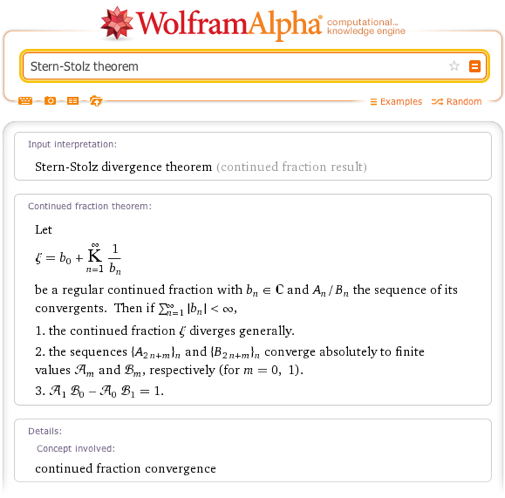The results returned for these queries contain human-readable forms of the theorems, references, and some information about the people who investigated them. The various result types are hyperlinked to the extent possible (e.g., Queffelec’s theorem deals with regular continued fractions and with the Thue–Morse sequence).

Although the Stern–Stolz theorem is a classic and important theorem in the theory of convergence of continued fractions, trying a Google Scholar search for Stern–Stolz theorem will show a few dozen results, and one has to read (or at least scan) the first few in order to find out what the Stern–Stolz theorem actually is. Clearly, having immediate access to the distilled version of the theorem is efficient. To make the theorems more easily and quickly understandable, and also easier to compare, we aimed for two canonicalizations:

a) Explanation of all symbols occurring in a theorem.

In the original papers, often authors first introduce some notation and then use this notation later in a theorem. So to understand the theorem, one often cannot just locally read the theorem, but must first locate the relevant notations.

b) Usage of uniform notations as much as possible.

The terms of a continued fraction [b1/a1, b2/a2, …] are often denoted by ak and bk. But which one is the denominator and which one is the numerator depends on the continued fraction investigated. For example, the bj are the denominators in a general continued fraction, but in a regular continued fraction they are often denoted aj. Similarly, numerators and denominators of convergents are commonly denoted pj and qj in the historical literature, but Ak and Bk in more recent papers and books. In the theorems collected within this project, we canonicalized most of the notations to make looking at more than one theorem at a time less ambiguous.

Some important theorems are encapsulated in concrete formulas: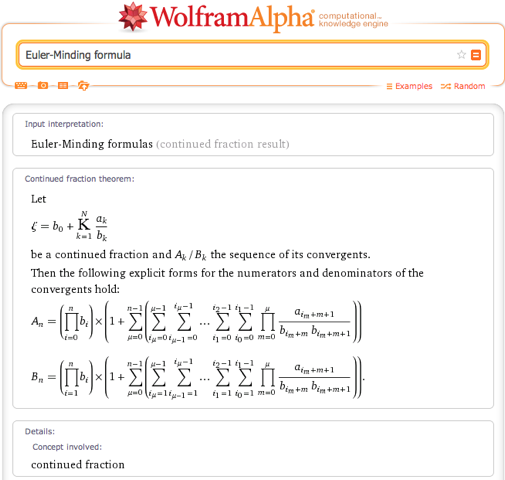And here are two named conjectures about continued fractions: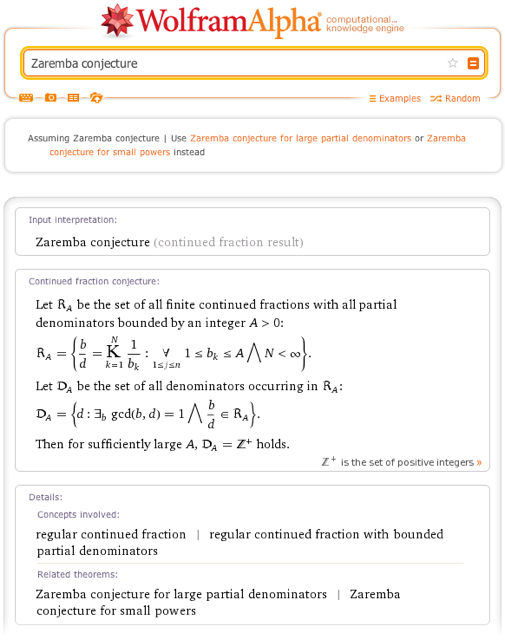The majority of mathematical theorems are not prominent enough to have been explicitly named after their provers, so finding a standard or accepted name by which to refer to them can be tricky. Our implementation partially solves this problem in the case where known people are associated with a given result:

Of course, in most real-life research, one is commonly interested in finding mathematical theorems about a certain topic/concept, not in looking up a theorem by name. As a result, we also allow searching by topic:

Special classes of mathematical structures often allow for more detailed investigations. For instance, quadratic irrationals have periodic regular continued fractions expansions, and many special theorems hold for quadratic irrationals. So, one can also query for theorems that hold for certain classes of mathematical objects:

Having the theorems inside a computational system, we can utilize the computational system and check if the conditions of a certain theorem hold in concrete instances: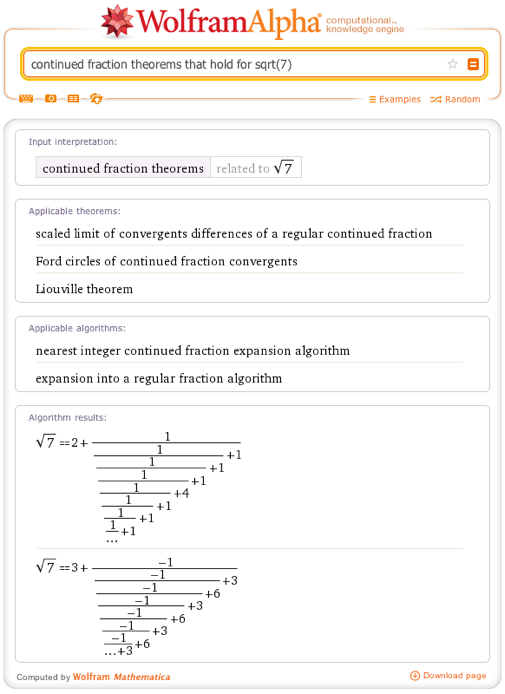Sometimes theorems are generalizations of earlier found theorems or special cases of more general theorems. Or theorems are related to each other in the sense that they deal with quite related topics. As much as our limited theorem subset allowed such connections, one can query for them:

Finally, linking the theorems about continued fractions to other Wolfram|Alpha databases, such as the people database, enriches the results and literally puts a face on a certain theorem: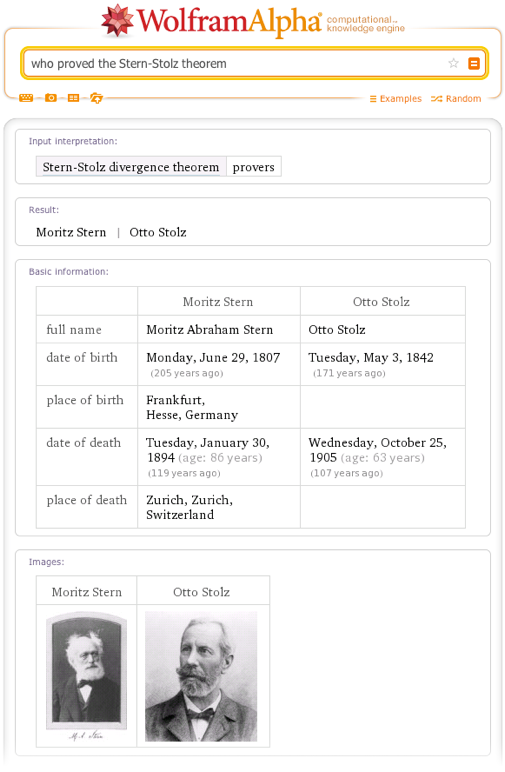### Mathematical Identities

While there are many integral tables (Oleg Marichev, co-author of the comprehensive five-volume Integrals and Series handbook, was a team member on this continued fraction project) and tables for sums, surprisingly there is no published comprehensive table for continued fractions. By carrying out a comprehensive literature search, we attempted to collect all known continued fraction representations. (If the reader knows of a continued fraction that we don’t have, please let us know and we would be happy to add it to our collection.) Some examples include: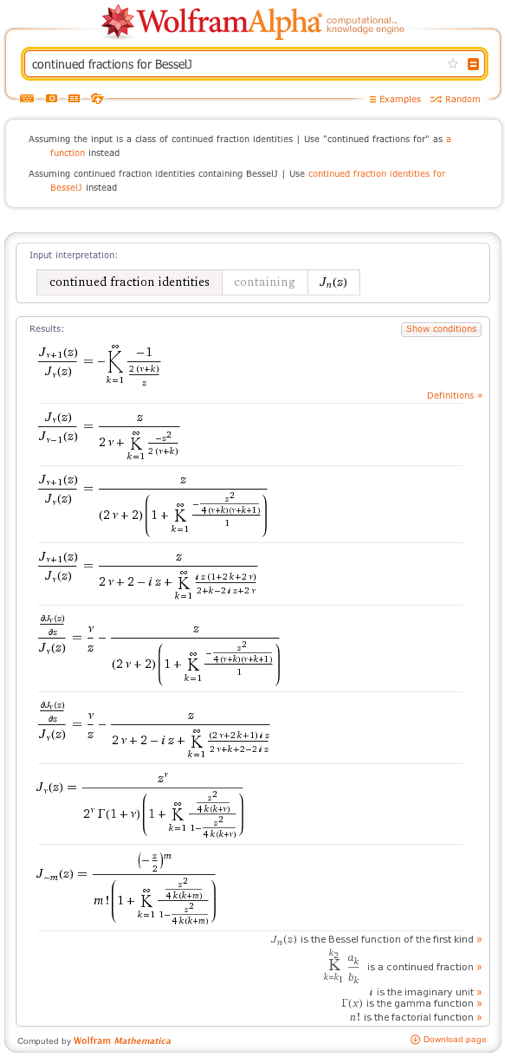As an example of issues encountered and resolved in the curation of continued fraction identities, we note that the older literature did not always pay detailed attention to branch cut issues. While one can in principle use any branch cut location that leads to “nice” identities, this nonstandard treatment should be banished in the modern world in favor of the precisely defined IEEE 754 specifications of branch cuts (section 9.2), as is done for example in Mathematica. In our curated identities, the branch cuts were carefully checked and standardized and simplify appropriately in all relevant regions of the complex plane.

To use these identities in programs, one can either utilize the copyable plaintext feature on Wolfram|Alpha or the WolframAlpha function in Mathematica. For instance, the following table summarizes the relative error for the continued fractions of π when truncating the expansion at 10 continued fraction terms: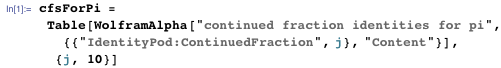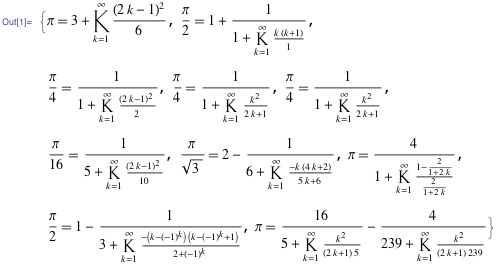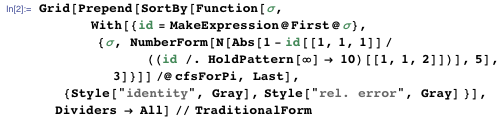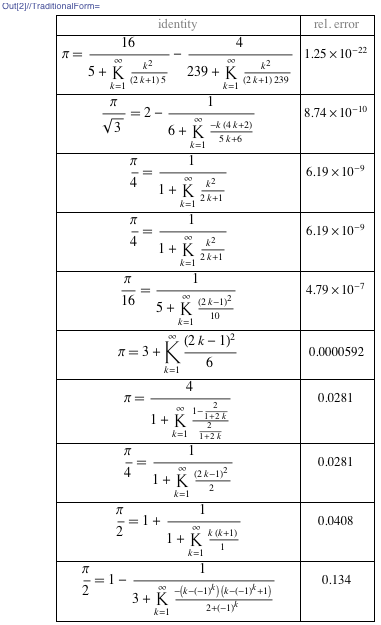### Definitions and Concepts

In addition to theorems, we need appropriate phrases to define and describe various continued fraction topics. For instance, the most appropriate convergence concept for continued fractions is different from the convergence for series: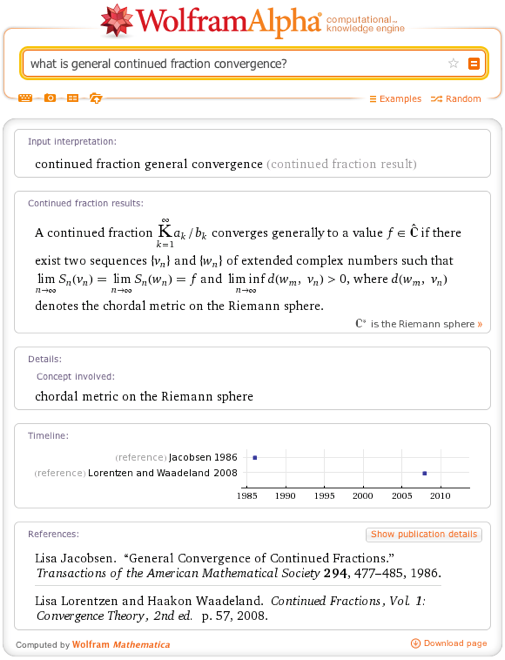There are many different types of continued fractions that contain indeterminates:

And various operators and operations relevant for continued fractions:

Given a real number, the most straightforward way to build a continued fraction expansion is the regular continued fraction expansion. But there are many other ways to build continued fraction expansions for a given real number:

### Algorithms

While Wolfram|Alpha uses a tremendous number of algorithms to answer a large variety of queries, they are all “under the hood.” Within our continued fraction project, the goal was to both implement the most important algorithms for continued fractions and to clearly and precisely describe and expose them: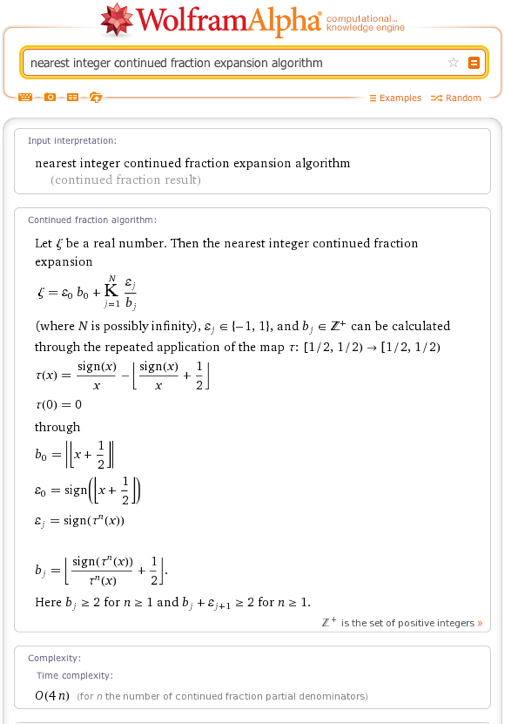One can also watch the algorithms “in action”:

### Visualizations and Interactive Demonstrations

Various continued fraction theorems that deal with the distribution of continued fraction digits hold for almost all real numbers. In many cases, the concrete numbers for which these theorems do not hold cannot be explicitly enumerated, so one is naturally curious if and how, as a function of the number of terms, the statements of these theorems hold for a concrete real number. To satisfy such curiosity and to stimulate further research, we added interactive exploration tools for a subset of the theorems:

One can also make other interactive explorations, for example by viewing the beautiful constellations of nested touching circles that arise in forming the convergents of Schmidt’s continued fractions for complex numbers: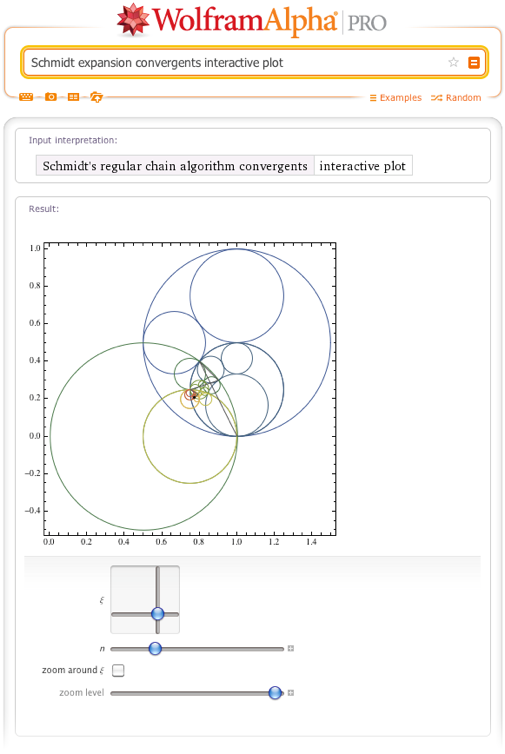### References

Mathematical Reviews and Zentralblatt offer excellent bibliographic data for an enormous number of mathematics papers. For completeness, we added cross-linking to these and other established mathematical literature sites to the papers and books present in our prototype. Within our implementation, references can be searched based on the journal in which a source paper appears:

Similarly, one can query for the papers due to a particular person:

## Conclusions

This ends our short overview of the work we have done to encode mathematical knowledge relevant to continued fractions as part of a grant from the Sloan Foundation.

We welcome any suggestions for changes and additions, as well as any improvements our users may suggest. In addition to adding various additional theorems, identities, and features, we will be working on extending and making our results more useful over the next months. The most complete tables of special values and modular equations for the Ramanujan continued fraction are examples of additional material that will be added soon; see the recent blog post on the subject for more details and a showcasing of some new results we found in assembling these tables.